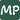# How to Calculate the Number of Months Between Two Dates in Javascript

November 05, 2021

Manipulating dates in Javascript is an extremely common requirement for a large number of apps and server logic (when using NodeJs). The ability to retrieve the number of months between dates is a very common and useful skill to have in your toolkit.

## Calculating The Number of Months

To do this, we need both the month and year components of the two dates. We then calculate the number of months between the month components and add the difference in years multiplied by 12, which converts the years to months.

``````const startDate = new Date(2020, 1, 12); // Feb 12th 2020
const endDate = new Date(2022, 4, 18); // April 18th 2022

const monthDiff = endDate.getMonth() - startDate.getMonth() +
(12 * (endDate.getFullYear() - startDate.getFullYear()));
console.log(monthDiff); // 27``````

This method does not take into account partial amounts of the month that have progressed between the two dates (in the case above the end date is 6 days ahead so the full amount would be approximately 27.2).

If you are unable to ascertain which is the start and end dates, wrapping the monthDiff calculation with Math.abs() will always return the positive value:

``````const dateOne = new Date(2020, 1, 12); // Feb 12th 2020
const dateTwo = new Date(2022, 4, 18); // April 18th 2022

const monthDiff = Math.abs(dateOne.getMonth() - dateTwo.getMonth() +
(12 * (dateOne.getFullYear() - dateTwo.getFullYear())));
console.log(monthDiff); // 27

const otherMonthDiff = Math.abs(dateTwo.getMonth() - dateOne.getMonth() +
(12 * (dateTwo.getFullYear() - dateOne.getFullYear())));
console.log(otherMonthDiff); // 27``````Blog by Mick Patterson